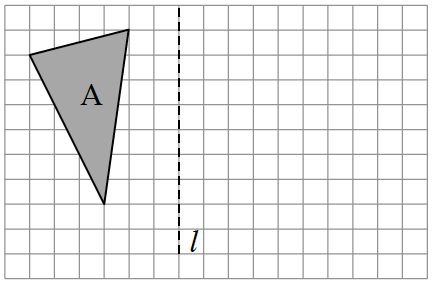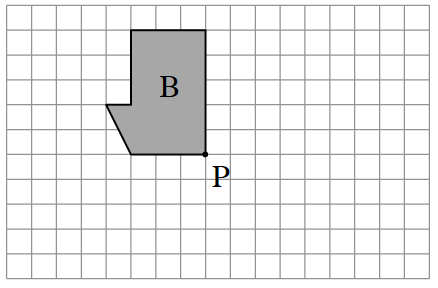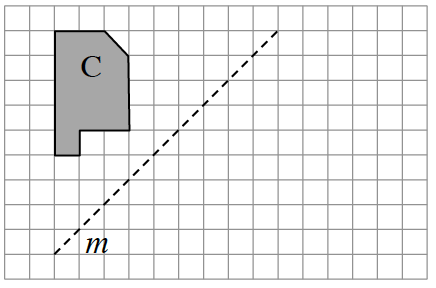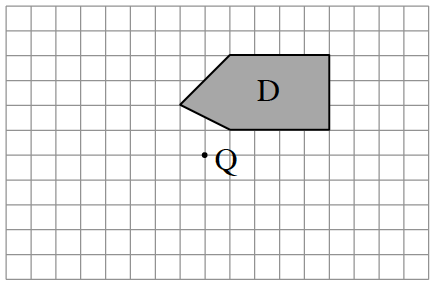### Home > CCG > Chapter 1 > Lesson 1.2.5 > Problem1-97

1-97.

Copy the diagrams below on graph paper. Then find the result when each indicated transformation is performed.

1. Reflect Figure $A$ across line $l$.1. Rotate Figure $B$ $90º$ clockwise ($\circlearrowright$) about point $P$.1. Reflect Figure $C$ across line $m$.1. Rotate Figure $D$ $180º$ about point $Q$.The eTool below shows the transformations for all parts.
Click the link at right for the full version of the eTool: 1-97 HW eTool